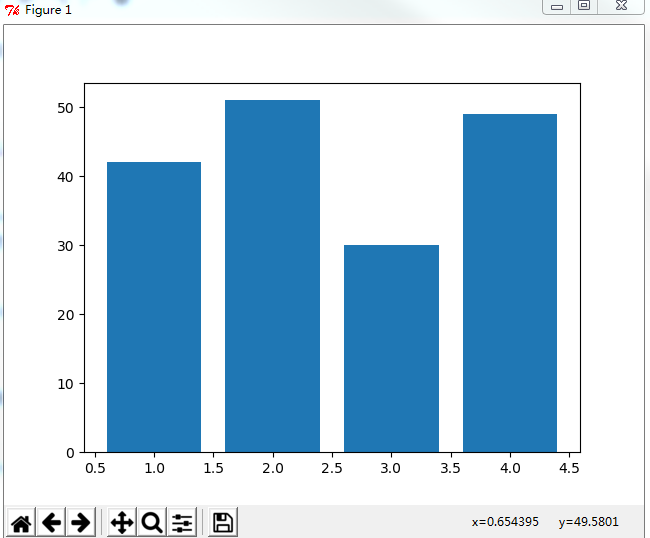## matplotlib使用：画柱状图

matplotlib画柱状图十分简单，首先需要导入matplotlib中的pyplot，这个就是能够画图的类啦

`import matplotlib.pyplot as plt`

```students = [42,51,30,49]
x = [1,2,3,4]```

```plt.bar(x=x, height=students)
plt.show()```

```#coding:utf-8
import matplotlib.pyplot as plt

students = [42,51,30,49]
x = [1,2,3,4]

plt.bar(x=x, height=students)
plt.show()
```